# How to Calculate and Solve for Resistance of a Temperature Detector | Temperature Measuring Instruments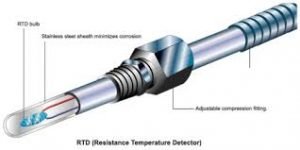The image above represents resistance of a temperature detector.

To compute for resistance of a temperature detector, four essential parameters are needed and these parameters are Reference Resistance (Ro), Temperature Co-efficient of Resistivity (α), Temperature of Thermistor (T) and Ambient Temperature (To).

The formula for calculating resistance of a temperature detector:

R = Ro [1 + α(T – To)]

Where:

R = Resistance
Ro = Reference Resistance
α = Temperature Co-efficient of Resistivity
T = Temperature of Thermistor
To = Ambient Temperature

Let’s solve an example:
Find the resistance when the reference resistance is 11, the temperature co-efficient of resistivity is 13, the temperature of thermistor is 17 and the ambient temperature is 19.

This implies that;

Ro = Reference Resistance = 11
α = Temperature Co-efficient of Resistivity = 13
T = Temperature of Thermistor = 17
To = Ambient Temperature = 19

R = Ro [1 + α(T – To)]
R = 11 x [1 + 13 x (17 – 19)]
R = 11 x [1 + 13 x (-2)]
R = 11 x [1 + -26]
R = 11 x -25
R = – 275

Therefore, the resistance of temperature detector is – 275 Ω.

Nickzom Calculator – The Calculator Encyclopedia is capable of calculating the resistance of a temperature detector.

To get the answer and workings of the resistance of a temperature detector using the Nickzom Calculator – The Calculator Encyclopedia. First, you need to obtain the app.

You can get this app via any of these means:

To get access to the professional version via web, you need to register and subscribe for NGN 2,000 per annum to have utter access to all functionalities.
You can also try the demo version via https://www.nickzom.org/calculator

Apple (Paid) – https://itunes.apple.com/us/app/nickzom-calculator/id1331162702?mt=8
Once, you have obtained the calculator encyclopedia app, proceed to the Calculator Map, then click on Materials and Metallurgical under Engineering.Now, Click on instrumentation under Materials and MetallurgicalNow, Click on Temperature Measuring Instruments under Instrumentation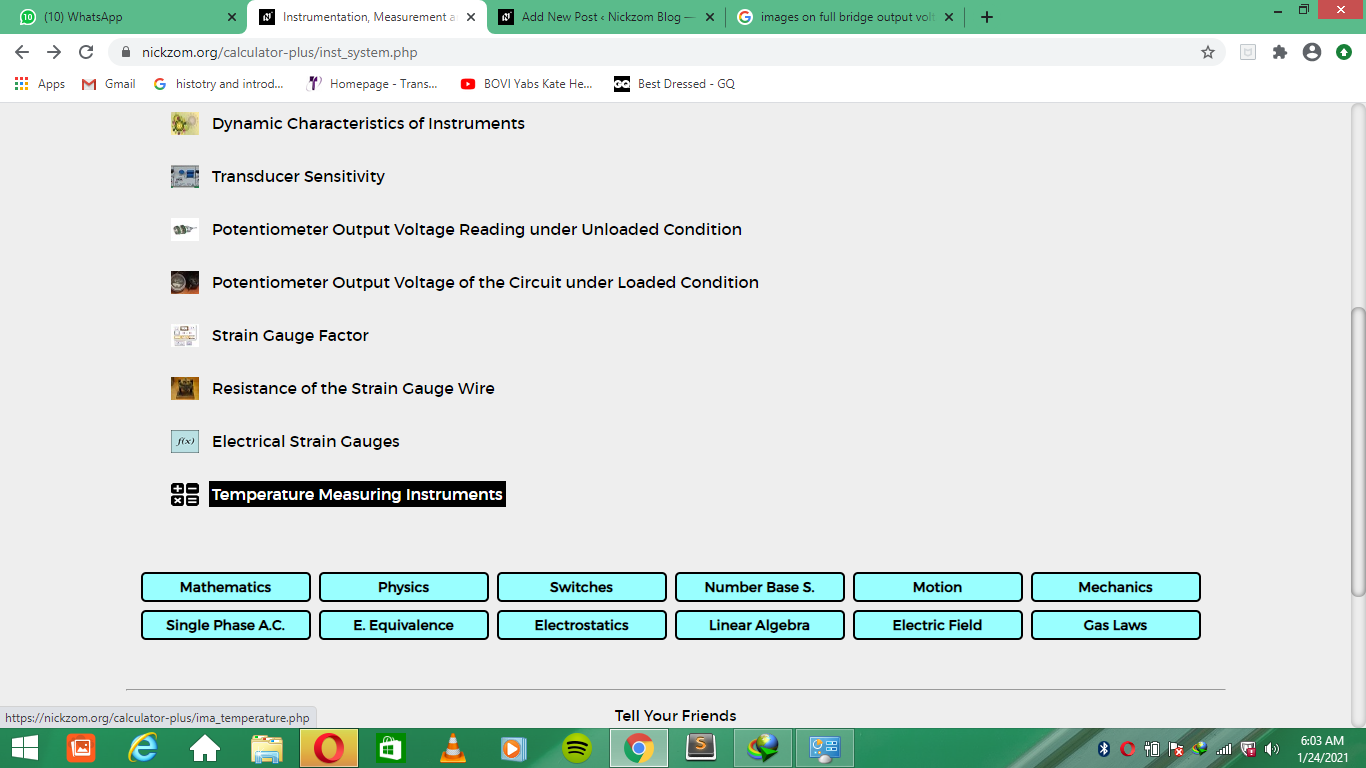Now, Click on Resistance of a Temperature Detector under Temperature of Measuring Instruments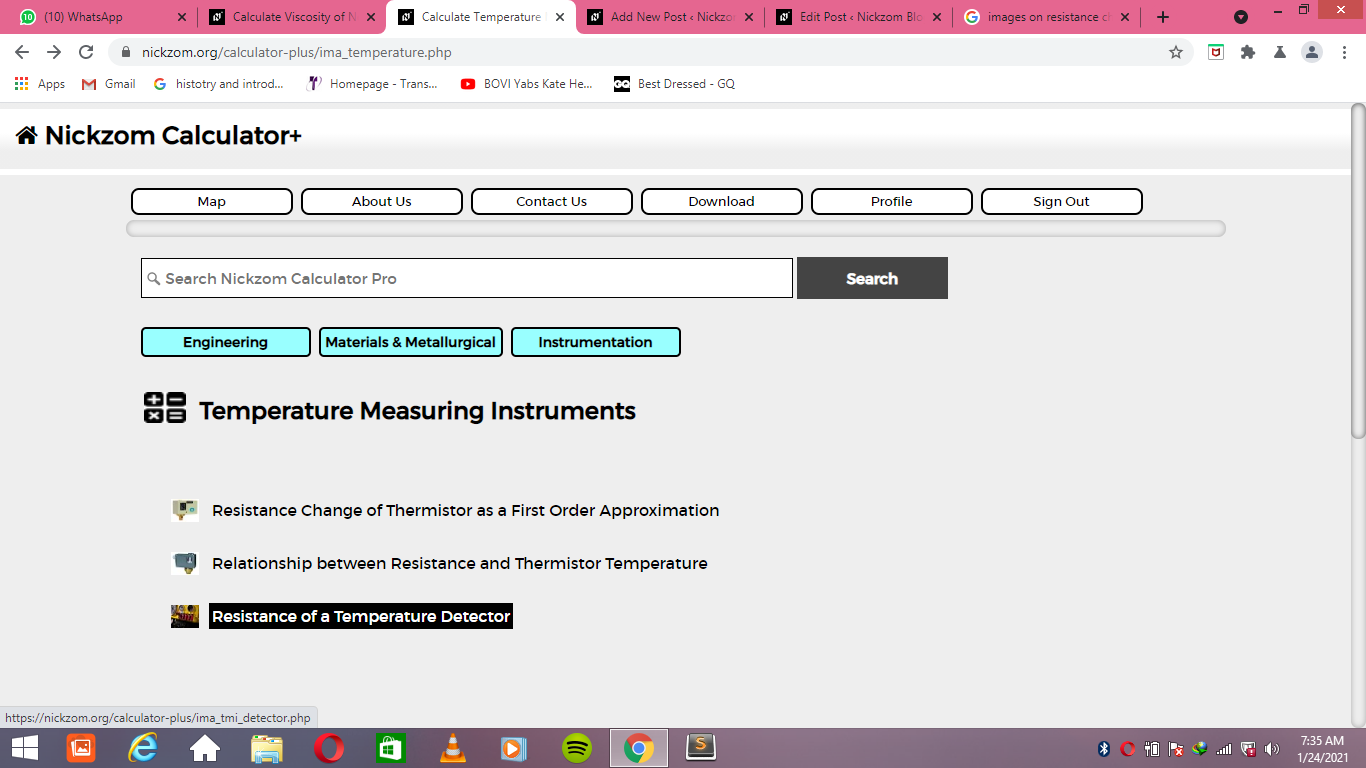The screenshot below displays the page or activity to enter your values, to get the answer for the resistance of a temperature detector according to the respective parameters which is the Reference Resistance (Ro), Temperature Co-efficient of Resistivity (α), Temperature of Thermistor (T) and Ambient Temperature (To).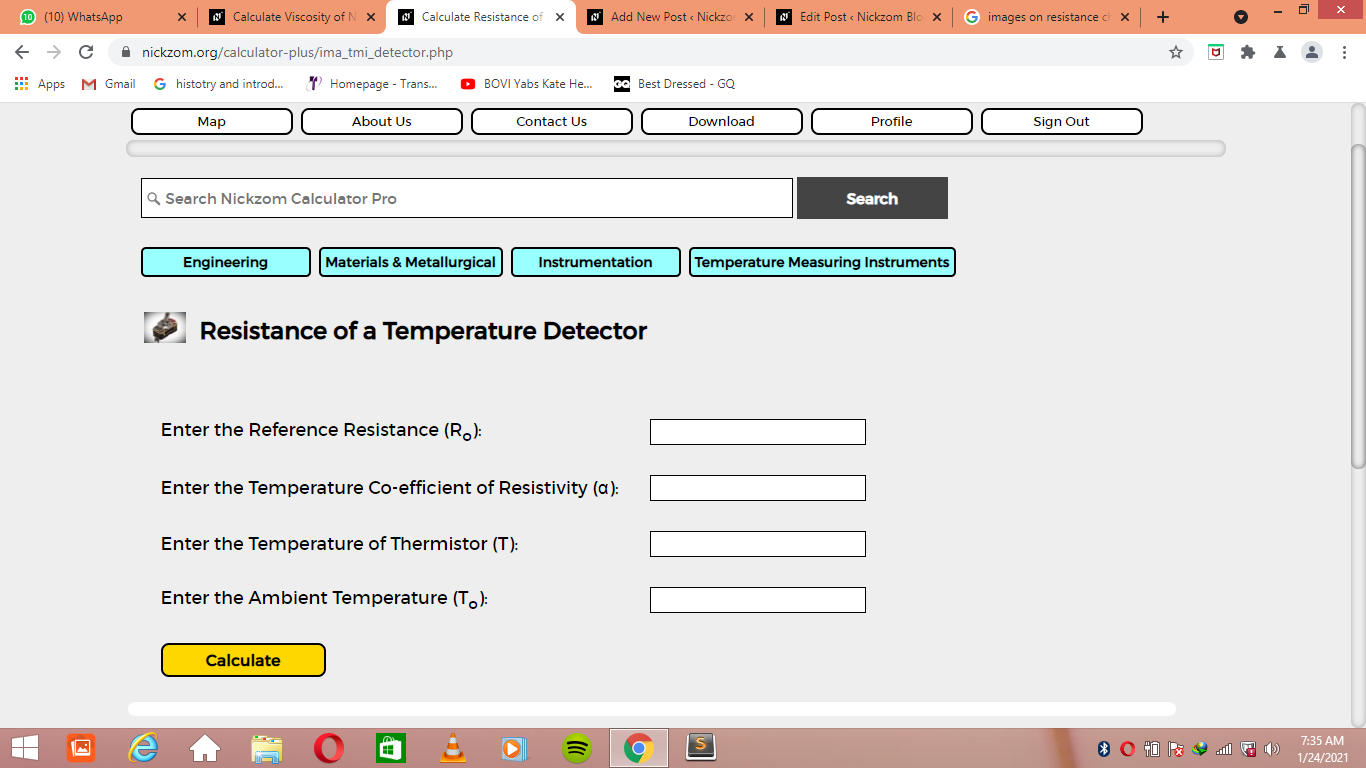Now, enter the values appropriately and accordingly for the parameters as required by the Reference Resistance (Ro) is 11, Temperature Co-efficient of Resistivity (α) is 13, Temperature of Thermistor (T) is 17 and Ambient Temperature (To) is 19.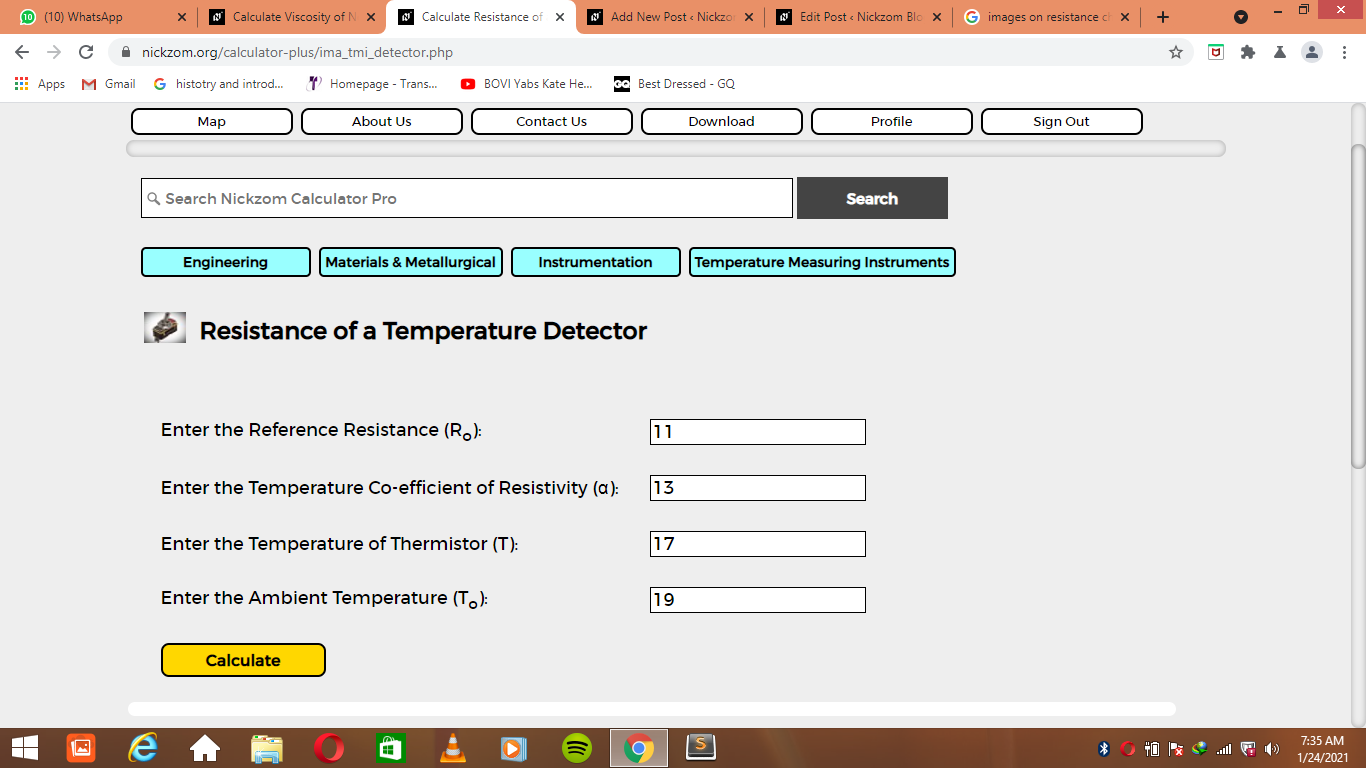Finally, Click on Calculate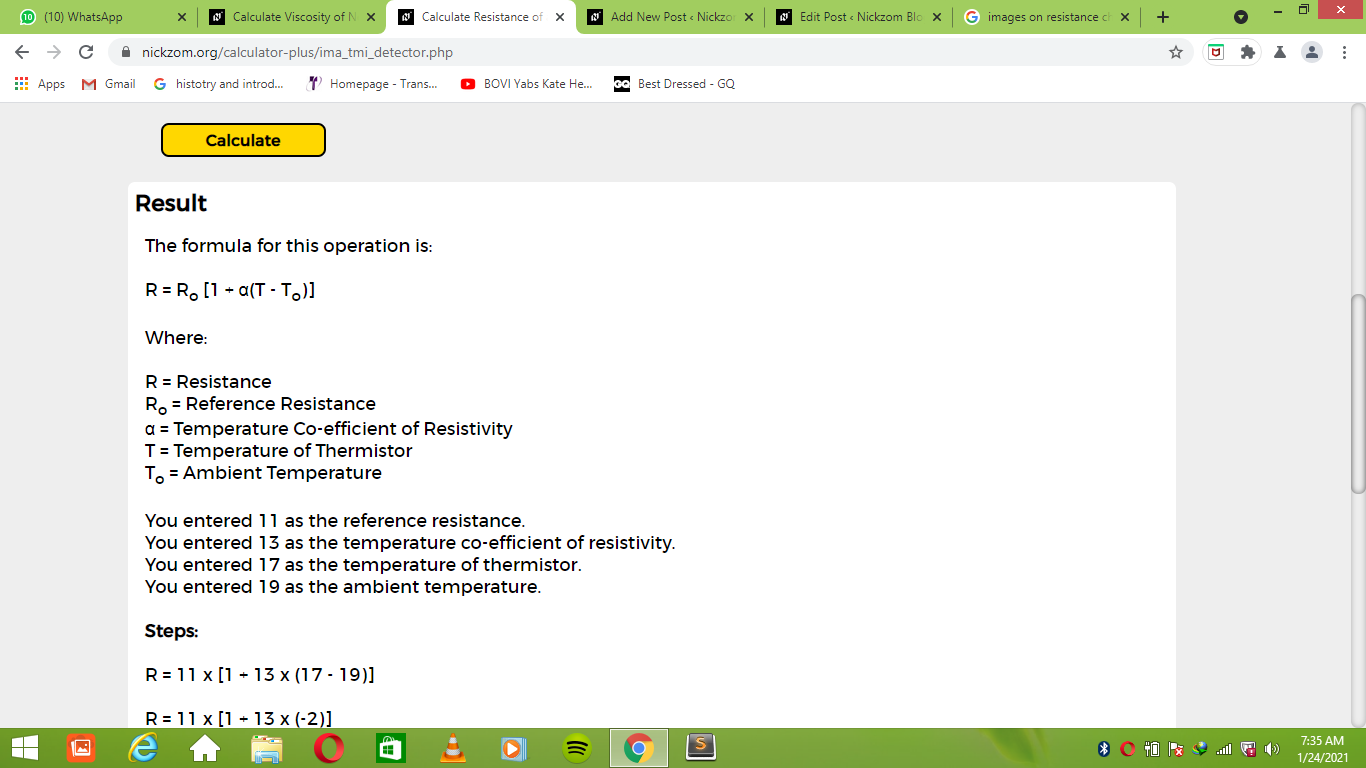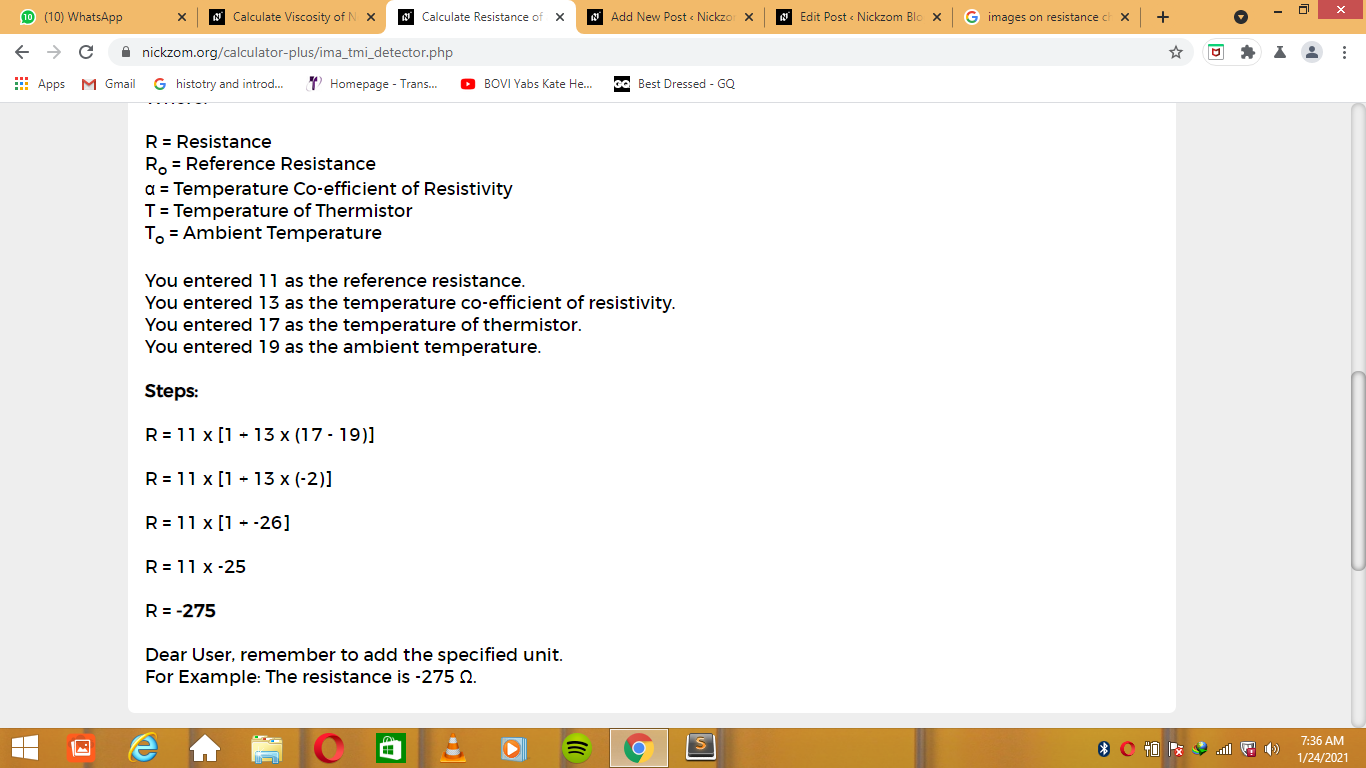As you can see from the screenshot above, Nickzom Calculator– The Calculator Encyclopedia solves for the resistance of a temperature detector and presents the formula, workings and steps too